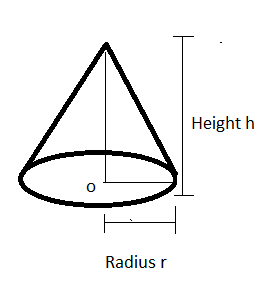QuestionAnswers

# Two cones have their heights in the ratio 1:3 and their radii of their bases in the ratio 3:1. Find the ratio of their volumes.Hint- In order to solve to this problem, we must choose formula of volume of cone which is equal to $\dfrac{1}{3}(\pi \times {{\text{r}}^2} \times {\text{h)}}$ along with the proper understanding of the figure that is being provided in the question.Here in this question it is given the ratios of heights and bases radii of two different cones and we have to find the volume ratio.
We know that, the volume of cone is $\dfrac{1}{3}(\pi \times {{\text{r}}^2} \times {\text{h)}}$ ……………………………... (1)
Let the radius, height and Volume of 1st cone be ${{\text{r}}_{1{\text{ }}}}{\text{, }}{{\text{h}}_1}{\text{ and }}{{\text{v}}_1}$ respectively
And
The radius, height and Volume of 2nd cone be ${{\text{r}}_{{\text{2 }}}}{\text{, }}{{\text{h}}_2}{\text{ and }}{{\text{v}}_2}$ respectively
So Using expression (1)
Volume of 1st cone ${{\text{v}}_1}{\text{ will be }}\dfrac{1}{3}(\pi \times {{\text{r}}_1}^2 \times {{\text{h}}_1}{\text{)}}$ …………………………………...(2)
And volume of 2nd cone ${{\text{v}}_2}{\text{ will be }}\dfrac{1}{3}(\pi \times {{\text{r}}_2}^2 \times {{\text{h}}_2}{\text{)}}$ …………………………………….(3)
Now on dividing equation (2) by (3),
${\text{Hence }}\dfrac{{{{\text{v}}_1}}}{{{{\text{v}}_2}}} = \dfrac{{\dfrac{1}{3}(\pi \times {{\text{r}}_1}^2 \times {{\text{h}}_1}{\text{)}}}}{{\dfrac{1}{3}(\pi \times {{\text{r}}_2}^2 \times {{\text{h}}_2}{\text{)}}}}$

On cancelling $\dfrac{1}{3}$ and $\pi$ from numerator and denominator,
$\therefore \dfrac{{{{\text{v}}_1}}}{{{{\text{v}}_2}}} = \dfrac{{({{\text{r}}_1}^2 \times {{\text{h}}_1}{\text{)}}}}{{({{\text{r}}_2}^2 \times {{\text{h}}_2}{\text{)}}}}$
$\therefore \dfrac{{{{\text{v}}_1}}}{{{{\text{v}}_2}}} = {\left( {\dfrac{{{{\text{r}}_1}}}{{{{\text{r}}_2}}}} \right)^2} \times \left( {\dfrac{{{{\text{h}}_1}}}{{{{\text{h}}_2}}}} \right)$ ………………………………….(4)
In the given question it is given that
$\therefore \left( {\dfrac{{{{\text{r}}_1}}}{{{{\text{r}}_2}}}} \right) =$ $\dfrac{3}{1}$ And $\left( {\dfrac{{{{\text{h}}_1}}}{{{{\text{h}}_2}}}} \right) =$$\dfrac{1}{3}$
Now putting the value of $\left( {\dfrac{{{{\text{r}}_1}}}{{{{\text{r}}_2}}}} \right)$ and $\left( {\dfrac{{{{\text{h}}_1}}}{{{{\text{h}}_2}}}} \right)$ in equation (4)
$\therefore \dfrac{{{{\text{v}}_1}}}{{{{\text{v}}_2}}}$ $= {\left( {\dfrac{3}{1}} \right)^2} \times \left( {\dfrac{1}{3}} \right)$
On solving
$\therefore \dfrac{{{{\text{v}}_1}}}{{{{\text{v}}_2}}} =$ $3$
Hence, the volume ratio of given cones will be $\dfrac{3}{1}$

Note: -Whenever we face such types of problems the key concept we have to remember is that always remember the formula of volume of cone which is stated above, then using this formula calculate the ratio of volume of two cones and simplify, we will get the required answer.
View Notes
Why do we Have Two Eyes?Physical Properties of Alkanes and Their VariationsExcretory Products and Their EliminationCBSE Class 9 Maths Chapter 13 - Surface Areas and Volumes FormulasCBSE Class 6 Maths Chapter 12 - Ratio and Proportion FormulasRatio and Proportion FormulaTable of 13 - Multiplication Table of 13CBSE Class 10 Maths Chapter 3 - Pair of Linear Equations in Two Variables FormulaCBSE Class 9 Maths Chapter 6 - Lines and Angles FormulasCBSE Class 8 Maths Chapter 13 - Direct and Inverse Proportions FormulasImportant Questions for CBSE Class 9 Maths Chapter 13 - Surface Areas and VolumesNCERT Books for Class 9 Maths Chapter 13Important Questions for CBSE Class 9 Science Chapter 13 - Why Do We Fall illImportant Questions for CBSE Class 6 Science Chapter 9 - The Living Organisms and Their SurroundingsNCERT Books for Class 9 Science Chapter 13CBSE Class 6 Science The Living Organisms and Their Surroundings WorksheetsImportant Questions for CBSE Class 9 Maths Chapter 4 - Linear Equations in Two VariablesImportant Questions for CBSE Class 6 Maths Chapter 12 - Ratio and ProportionImportant Questions for CBSE Class 6 English A Pact with The Sun Chapter 1 - A Tale of Two BirdsImportant Questions for CBSE Class 9 Maths Chapter 9 - Areas of Parallelograms and TrianglesCBSE Class 12 Maths Question Paper 2020CBSE Class 10 Hindi A Question Paper 2020Hindi A Class 10 CBSE Question Paper 2009Hindi A Class 10 CBSE Question Paper 2015Hindi A Class 10 CBSE Question Paper 2007Hindi A Class 10 CBSE Question Paper 2013CBSE Class 10 Maths Question Paper 2020Hindi A Class 10 CBSE Question Paper 2016Hindi A Class 10 CBSE Question Paper 2012Hindi A Class 10 CBSE Question Paper 2010RD Sharma Solutions for Class 9 Maths Chapter 20 - Surface Area and Volume of A Right Circular ConeRD Sharma Solutions for Class 9 Maths Chapter 13 - Linear Equations in Two VariablesNCERT Solutions for Class 9 Maths Chapter 13 Surface Areas and Volumes (Ex 13.9) Exercise 13.9RD Sharma Solutions for Class 9 Maths Chapter 19 - Surface Area and Volume of A Right Circular CylinderRD Sharma Class 9 Solutions Chapter 13 - Linear Equation In Two Variables (Ex 13.3) Exercise 13.3RD Sharma Class 9 Solutions Chapter 13 - Linear Equation In Two Variables (Ex 13.2) Exercise 13.2NCERT Solutions for Class 9 Maths Chapter 13 Surface Areas And Volumes (Ex 13.7) Exercise 13.7NCERT Solutions for Class 9 Maths Chapter 13 Surface Area and Volumes In HindiRD Sharma Class 9 Solutions Chapter 13 - Linear Equation In Two Variables (Ex 13.1) Exercise 13.1RD Sharma Class 9 Solutions Chapter 13 - Linear Equation In Two Variables (Ex 13.4) Exercise 13.4